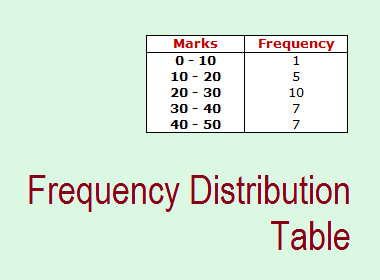# Frequency Distribution TableThe frequency is the number of times a data has been repeated. So the table which contains frequency and data is called a frequency distribution table or simply a frequency table.

## Frequency Table For Discrete Data:

Example: Let’s observe the marks secured by 20 students in a math test of 20 marks.

10, 8, 9, 8, 10, 10, 11, 12, 14, 15, 8, 9, 7, 8, 10, 10, 11, 12, 10, 8

The frequency distribution table of the above data is given below:

 Marks Frequency 7 8 9 10 11 12 14 15 1 5 2 6 2 2 1 1 N = 20

## Frequency Table For Group (Continuous) Data:

When the number of observations is a large amount of data which are converted into a compact form, we organize it in groups. These groups are called class intervals. We should make groups or classes of the same size. The difference between the upper limit and lower limit of a group is called class size.

Consider the following data showing the marks of 30 students in the first term examination.

23, 29, 40, 15, 30, 35, 45, 48, 25, 5, 16, 22, 18, 33, 42, 46, 23, 36, 31, 26, 24, 37, 42, 20, 19, 41, 28, 38, 29, 17

 Marks Frequency 0 - 10 10 - 20 20 - 30 30 - 40 40 - 50 1 5 10 7 7 N = 30

## Frequency Distribution Table For a Large Number of Data

For a large number of data, to draw a frequency table, we should use the following steps:

Step 1: Identify the smallest data and largest data.

Step 2: Divide the data into an appropriate class interval of the same size

Step 3: Common data always belongs to the higher class e.g. in 10-20 and 20-30, 20 lies in 20-30.

Step 4: Count the data and write tally bars in a frequency table.

## Cumulative frequency table

Cumulative frequency is a continuous frequency distribution in which frequencies are cumulated either in ascending or descending order. Look at the following example to be more clear about the cumulative frequency.

 Marks 10 20 30 40 50 60 No. of students 2 5 4 7 3 6

Cumulative frequency table:

 Marks No of students (f) Cumulative frequency (C.F.) 10 20 30 40 50 60 2 5 7 4 3 6 2 2+5=7 7+7=14 14+4=18 18+3=21 21+6=27 N = 27

From the above table, it is clear that the cumulative frequency is the sum of the frequencies up to the corresponding class.

********************

********************

## Workout Examples

Example 1: Represent the following data in a frequency distribution table.

15, 10, 16, 9, 7, 8, 9, 10, 15, 10, 8, 4, 7, 8

Solution:

Here,

Frequency distribution table,

 Data Frequency 4 7 8 9 10 15 16 1 2 3 2 3 2 1 N = 14

Example 2: Represent the following data in a frequency distribution table by making appropriate class intervals.

4, 7, 12, 15, 50, 22, 25, 27, 29, 33, 39, 44, 47, 18, 51, 31, 20, 21, 41, 36

Solution:

Here,

Frequency distribution table,

 Data Frequency 0-10 10-20 20-30 30-40 40-50 50-60 2 3 6 4 3 2 N = 20

Example 3: Represent the following data in a cumulative frequency distribution table.

8, 7, 9, 16, 10, 10, 12, 15, 15, 12, 8, 7, 10, 10, 10, 12, 12, 14, 15, 18

Solution:

Here,

Cumulative frequency distribution table,

 Data Frequency C.F. 7 8 9 10 12 14 15 16 18 2 2 1 5 4 1 3 1 1 2 2+2=4 4+1=5 5+5=10 10+4=14 14+1=15 15+3=18 18+1=19 19+1=20 N = 20

You can use the comment box below to ask your questions or problems regarding the frequency distribution table here.